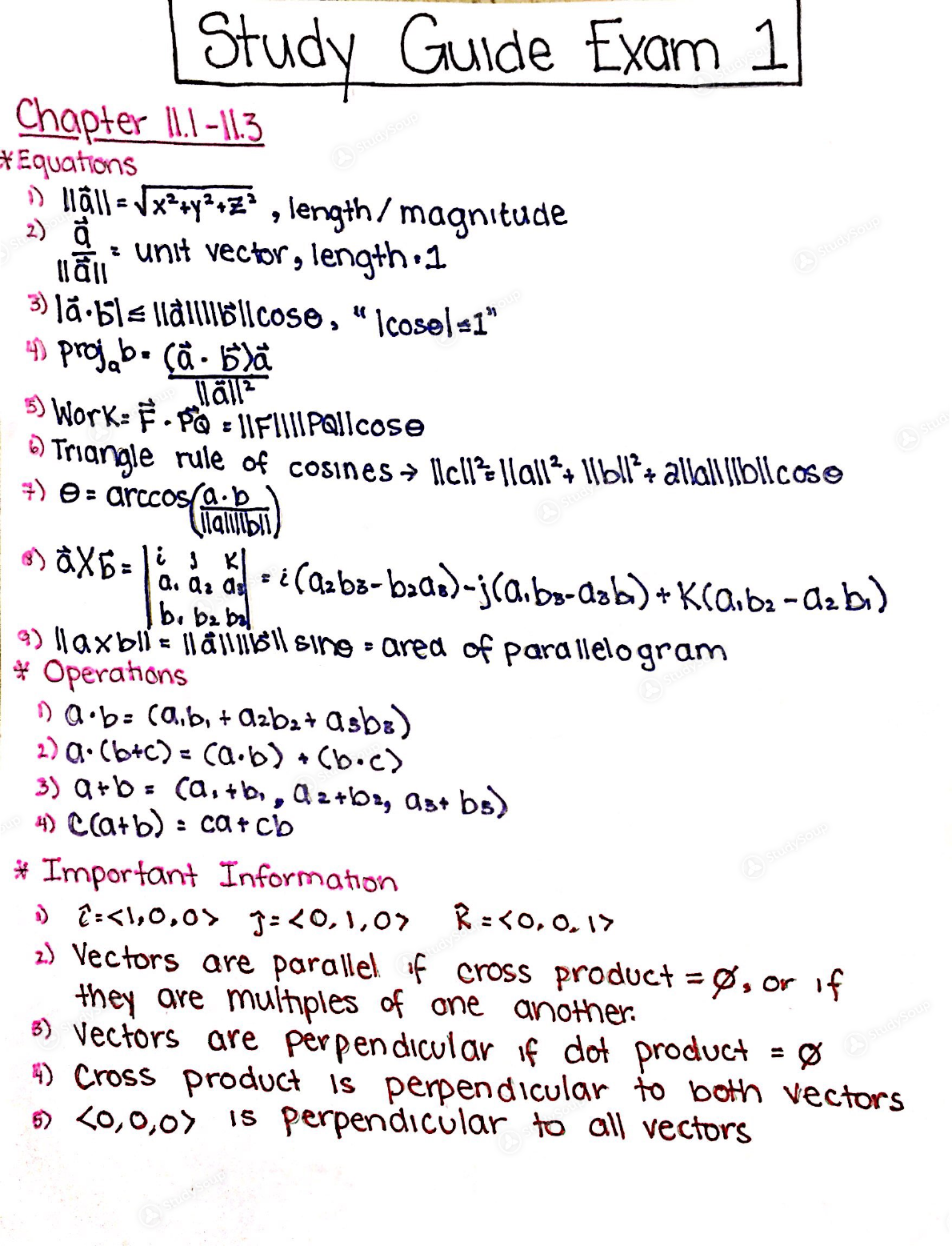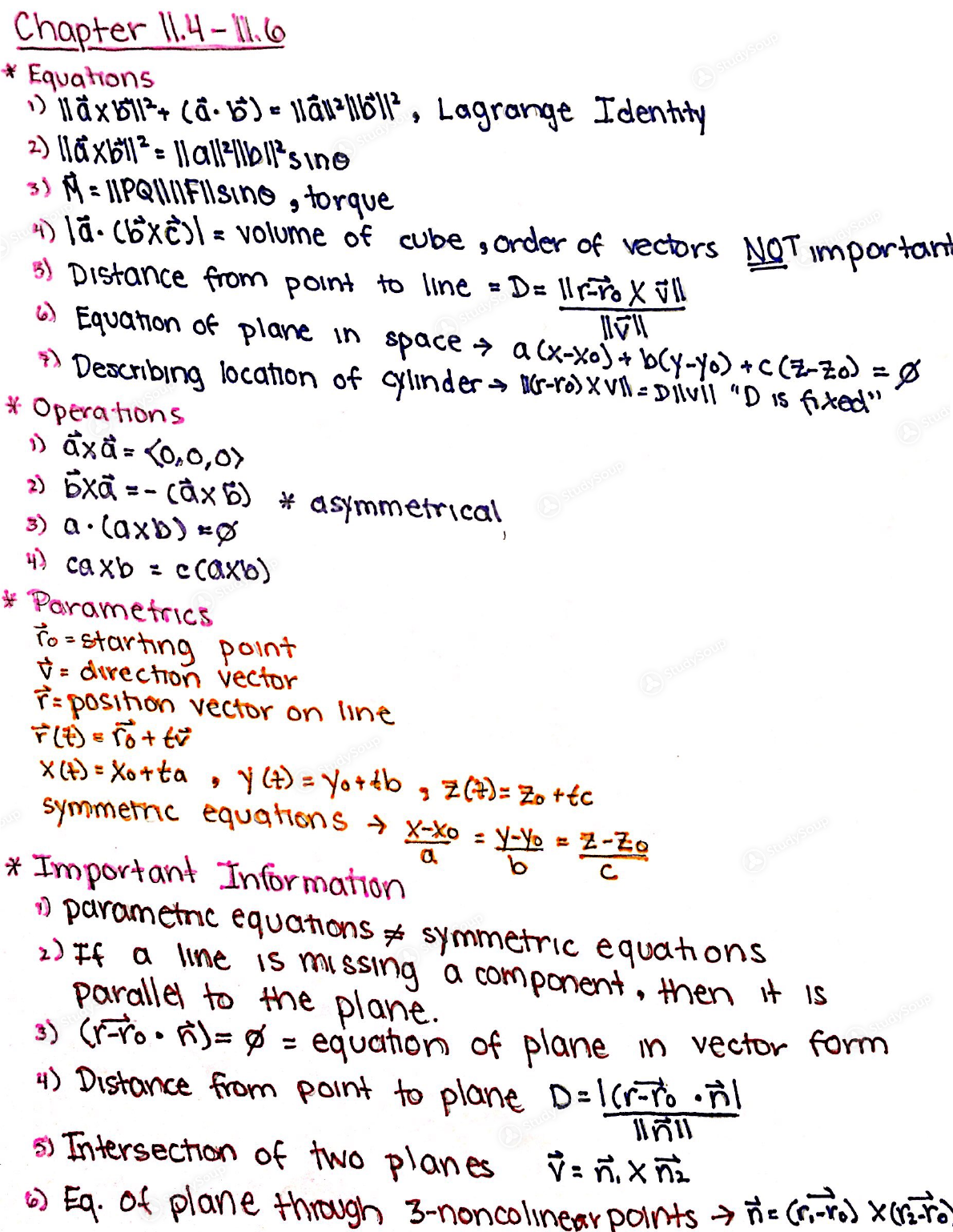Limited time offer 20% OFF StudySoup Subscription details

# MATH 241 - Study Guide

### Created by: Maya Silver-Isenstadt Elite Notetaker

> > > > MATH 241 - Study Guide

MATH 241 - Study Guide

0 5 3 92 Reviews
This preview shows pages 1 - 2 of a 2 page document. to view the rest of the contentScanned by CamScannerScanned by CamScannerThis is the end of the preview. Please to view the rest of the contentJoin more than 18,000+ college students at University of Maryland - College Park who use StudySoup to get ahead
##### Description: This study guide covers chapters 11-12.3
2 Pages 48 Views 38 Unlocks
• Notes, Study Guides, Flashcards + More!

Unformatted text preview: Study Guide Exam 1 2) af Chapter 11 -11.3 * Equations n ll = Tx**y*+z , length/ magnitude llant unit vector, length 1 3) t ble Kalliblicose , " Icosel 1* 4) prj.b. (a. Fa 5) Works f. Pa IFIIPallcose Triangle rule of cosines Icillall+ Ilbul* + alkalijiblicose +) = arccosa.pl laun) 958X6 l'aide des i (@zbi-baar)-jca.be-arb) + Kca.ba -a2b) b. pr. bil 9) laxbils Habil sing ared of parallelogram + Operahons na.b: (a.b, +azbar asbe 2) a.(btc) = ca.b). Co.c) 3) a+b(a. tbr, d r, as+ bs) 4) Ca+b) : car clo * Important Information <,0,0> : <0,1,0, R = , 0, 1> 25 Vectors are parallelf cross product = they are mulhples of one , or if another. B) Vectors are perpendicular if dot product = \$ 4) Cross product is perpendicular to potin vectors Co,0,0Is perpendicular to all vectorsChapter 11.4-Il. * Equations > T XBII+ (- 6). 1&r=bbil', Lagronge Idenhty 2) 116x61 = Aaloisine 3) A = APQUFTIsino , torque Dla.coXC = volume of cube sorder of vectors NOT important 5) Distance from point to line =DE 1 - XVI U Equation of plane in space a(x-xo) + BCYCCS- Deanbing location of cylinder - Tr-ras XvheDiVit "D is fixed = * Opera hons Saxa=0,0,) 2) BX = - c x) * asymmetrical 3) a.laxb) = cax'D = ccaxto) * Parametrics io - starting point G= dtrechon vector i= posihon vector on line TO=+ tv X (A) = Xotta, y) = yotib, z)= Zotec symmemnc equations X-Xo = Y-% = 2-zo * Important Information parornetnc equations et symmetric equations 2) Tf a line is missing a component, Hent Parallel to the plane. is 3) Gr-co. )= @ = equahon of planem vector form 4) Distance from point to plane D-lor-ro il Intersection of two planes o Eq. of plane through 3-noncolinear polnis iG-TS XV-TDati Chopier 13,1-13.3 * Equahons 1) Position: r*) = x(4)r + yt)+zCta ) Veoaty: v(t) = x 2 + D + Z'CHE 3) Speed = llvll A) Accelera hon : A) a ay : d' = x)T + y"{4} 22/R 5) Ft) - F,A437 + falt)? e fa atj2 Fldt = Soc udt )r + ...etc A) F'(A) = \im FEI-F(ta) + typ t-to * Opera hons 1) (F *C)() > FC + 60t 2)EXGY) = F(H) XG (4) >> A[fAnta): 774 flt) to'la Fe) ) (844X LD) A) X2&t') + r. Met (4) * Important Information an integral is Not defined, you need a @ constant 2) To Ketch a wf,make a T-chart and plot- points 3) Parame mzahon is usually piece-wise

×

I don't want to reset my password

Need help? Contact support

Need an Account? Is not associated with an account
We're here to help